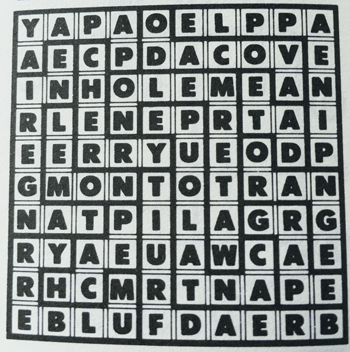Select Page

October 2016

Lots of Working Memory practice for you this month.

Here are the answers to the October 2016 Brain Practice:

Missing Elements#1 – Move down and to the right.
The difference between each successive pair of numbers increases by 1.
5 + 2 = 7,  7 + 3 = 10,  10 + 4 = 14,  14 + 5 =  19,  19 + 6 = 25

Subtract 6 to get the number below and between them.
(2 + 5) – 6 = 1 ,  (5 + 7) – 6 = 6,  (7 + 8) – 6 = 9
(1 + 9) – 6 = 4

e.g. (2 + 5) – 6 = 1. This calculation is at the top left of the triangle, with the solution – the 1 – situated between and below the 2 and the 5.

The second line then becomes (1 + 6) – 6 = 1  and this calculation is again placed between and below the 1 and the 6.

Same for (6 + 9) – 6 = 9  the result placed between and below.

Then the third line:  (1 + 9) – 6 = 4

What am I?

Riddle 1: A bar of soap

Riddle 2: Time

How to reach the other side?

Both of the boys go to the far side. One hops out and the other returns to the three men. The first man crosses alone, leaving one boy on each side. The boy on the side where the first man has been left then crosses back and picks up the other boy who then takes the first boy back to the other bank, drops him there and crosses back. The second man.crosses the river to where one boy is waiting. That boy crosses the river, picks up the other boy and they both cross back again, leaving one boy with the two men who have safely crossed. A rather tired boy then rows back to the other side the third tramper crosses safely. The waiting boy rows back to pick up his friend and some very grateful trampers carry on their way.

HAIRY, CHUTE, SHINGLE

RABBIT, TOTE, FILETS

TITLE, DETRER, COMETS

PAUNCH, GAIN, PALATE

FEVER, RIFLES, BELIEF

PEDAL, DANGLES, LODGE

Tangle Word – Have fund deciphering this!

Category: Fruit and vegetables.